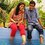# sharky's problem !!

some weeks ago sharky kesa posted a note that proves that 1+1=1 here is a better version of it $$\infty =\infty \\ { \infty }^{ 2 }=\infty \times \infty \\ { \infty }^{ 2 }-{ \infty }^{ 2 }=\infty \times \infty -{ \infty }^{ 2 }\\ (\infty +\infty )(\infty -\infty )=\infty (\infty -\infty )\\ cancelling\quad (\infty -\infty )\quad from\quad both\quad sides\\ and\quad we\quad got\quad \\ 2\infty =\infty \\ \infty =0\\ \frac { 1 }{ 0 } =0\\ 1=0\quad \\ here\quad in\quad place\quad of\quad 1\quad we\quad can\quad put\quad any\quad integer$$ hence shown that any integer can be equal to 0

note: i know that it is wrong but maths is fun !!Note by Rishabh Jain
7 years, 2 months ago

This discussion board is a place to discuss our Daily Challenges and the math and science related to those challenges. Explanations are more than just a solution — they should explain the steps and thinking strategies that you used to obtain the solution. Comments should further the discussion of math and science.

When posting on Brilliant:

• Use the emojis to react to an explanation, whether you're congratulating a job well done , or just really confused .
• Ask specific questions about the challenge or the steps in somebody's explanation. Well-posed questions can add a lot to the discussion, but posting "I don't understand!" doesn't help anyone.
• Try to contribute something new to the discussion, whether it is an extension, generalization or other idea related to the challenge.

MarkdownAppears as
*italics* or _italics_ italics
**bold** or __bold__ bold
- bulleted- list
• bulleted
• list
1. numbered2. list
1. numbered
2. list
Note: you must add a full line of space before and after lists for them to show up correctly
paragraph 1paragraph 2

paragraph 1

paragraph 2

[example link](https://brilliant.org)example link
> This is a quote
This is a quote
    # I indented these lines
# 4 spaces, and now they show
# up as a code block.

print "hello world"
# I indented these lines
# 4 spaces, and now they show
# up as a code block.

print "hello world"
MathAppears as
Remember to wrap math in $$ ... $$ or $ ... $ to ensure proper formatting.
2 \times 3 $2 \times 3$
2^{34} $2^{34}$
a_{i-1} $a_{i-1}$
\frac{2}{3} $\frac{2}{3}$
\sqrt{2} $\sqrt{2}$
\sum_{i=1}^3 $\sum_{i=1}^3$
\sin \theta $\sin \theta$
\boxed{123} $\boxed{123}$

Sort by:

The flaw is that when you divide by $(\infty-\infty)$ on both sides you divide by $0$. Cool! :D

- 7 years, 2 months ago

hey finn , i have already mentioned that it's wrong but thanks for your reply hey is there any e-mail id of you i have questions that are silly but i want to ask you if you can help me it would please me !!!

- 7 years, 2 months ago

@Finn Hulse I am not very sure,but I don't think so that $\infty-\infty = 0$ [Although I know that the proof is still wrong and we can't divide by $\infty-\infty$]

- 6 years, 10 months ago

That's true since there are different levels of infinity.

- 6 years, 10 months ago

But in this proof they're taken to be the same thing.

- 6 years, 10 months ago

I never even saw this until now! @Rishabh Jain

- 6 years, 11 months ago